Home / Tutorials / Arduino Tutorial / Using AD9833 Signal Generator with Arduino# Using AD9833 Signal Generator with Arduino

Ever wondered how you can create sine, square or triangular waves with Arduino? Apparently, there’s a way with the help of an integrated circuit package called AD9833. With it, you can now create waveforms for communications, instrumentation or other related projects. My tutorial on building your own Arduino signal generator after the jump.

The AD9833, a product of Analog Devices, is a low-power, programmable waveform generator. It is capable of producing sine, square or triangular waves with frequencies from 0 to 12.5 MHz.

It’s a 10-pin IC with pinout shown below: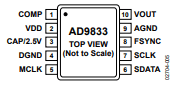This IC has a digital oscillator that produces a representation of a waveform which is then converted to an analog signal by a DAC. This circuit is also known as a direct digital synthesizer. The waveform is generated in the VOUT pin while the microcontroller interfaces with SPI pins SCLK and SDATA.

This function generator can run from a +5 V source although its internal circuitry runs on 2.5 V. The regulator that drops VDD voltage to 2.5 V requires a 100 nF external decoupling capacitor on the CAP/2.5 V pin. Moreover, the COMP pin is for decoupling the DAC bias voltage and thus also requires a 10 nF capacitor to ground.

The AD9833 communicates with microcontrollers via SPI. It has a control register for its configuration and frequency and phase registers for specifying the frequency and phase of the output waveform.

Note that there are two channels, thus, there is one frequency and phase registers for each channel.

The frequency output is calculated by using the formula: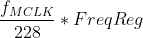Where fMCLK is equal to the crystal frequency on MCLK pin. This output frequency is then shifted to a phase given by:FreqReg (28-bit) and PhaseReg (12-bit) are data to be written to the frequency and phase registers.

Understanding phase and frequency in waveforms.

#### Control and Data Bits

For the AD9833 to operate, the microcontroller sends 16-bit words in frames. The state of the FSYNC pin acts as a frame for each 16-bit word, that is, the AD9833 can only read data when FSYNC is low.

Bits 15 and 14 determine the location of the register to which the data is written to. When these bits are both 0, data is sent to the control register. Otherwise, data is sent to other registers. See table below:The rest of the bits are either data (when in frequency or phase write) or other control bits.When writing to the control register, the rest of the bits are as follows:

B28 - if set, allows two successive frames as 14 MSBs and 14 LSBS.

HLB - if B28 is not set, the state of HLB determines if the received data is 14 MSB (HLB=1) or 14 LSB (HLB=0).

FSELECT - determines which channel to use for frequency

PSELECT - determines which channel to use for phase

RESET - resets internal registers but not the frequency, phase and control registers

SLEEP1, SLEEP12OPBITEN, DIV2, MODEYou cannot configure the AD9833 with just a single 16-bit word. A series of frames must be sent by the microcontroller to achieve the right configuration.

#### Example Data Transmission

Let’s have an example: a 25 MHz crystal attaches to the MCLK pin and your target is a 400 Hz sine wave at channel 0, without any phase shift. The value to be written to the frequency register is then:The first 16-bit word to be sent is for the control register. We also want the IC to reset. Hence, the 16 bits would be: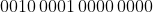Note that bit 13 (B28) is set because we want that successive frames be written immediately to the frequency register. Also notice that the OPBITEN, Mode and DIV2 bits are also zeroes, indicating that the output waveform is a sine wave.

The next word would be: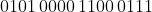Here, bit 15 and 14 points to the Freq0 register address (channel 0). The rest of the bits is the 14 MSB of the frequency we calculated earlier.

The next word:Still, bits 15 and 14 points to Freq0 and the rest of the bits are the 14 LSB of the frequency, which is now all zeroes.

Next we write to the phase register, even though it’s zero in this case: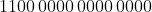Here, bits 15 and 14 points to Phase0.

Finally, we have:This writes to control register and issues a reset. A signal then appears at the output after seven MCLK cycles.

### Using the AD9833 with Arduino

To use the AD9833 with an Arduino, it’s better to buy a breakout board like this: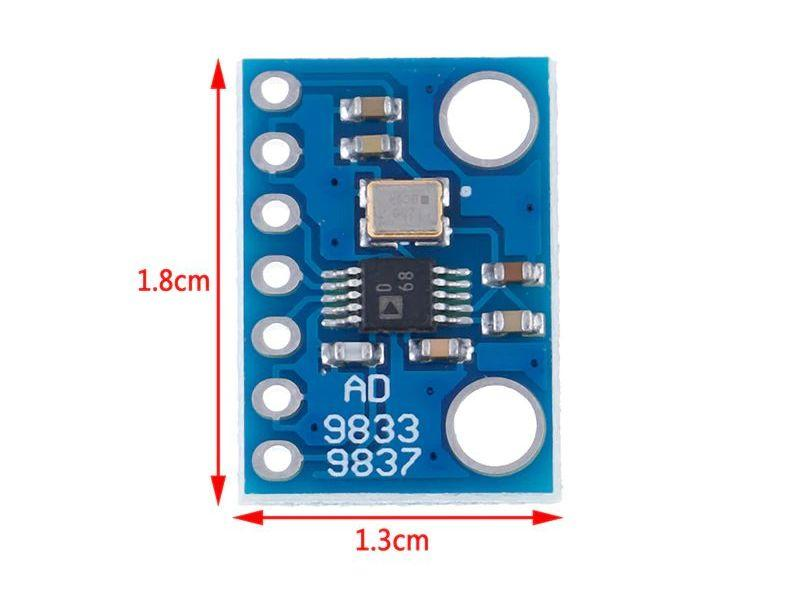Besides giving direct access to the AD9833 interface pins, this already has a crystal oscillator of 25 MHz and all the needed capacitors.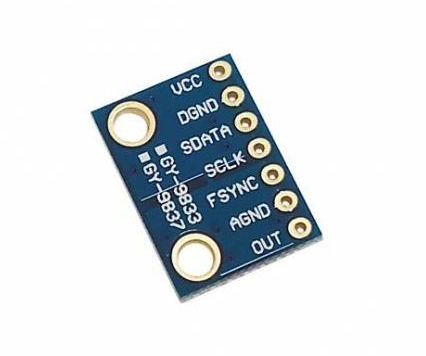#### Example Arduino Sketch

The library I recommend is that by Bill Williams. It uses the Arduino’s hardware SPI and so the breakout board connects to Arduino like this:The library has convenient functions for us to go away from all those bits and bytes. For example, producing a 1000 Hz sine wave only requires this code:

Here, the object initiates with:

FNC_PIN is user defined, meaning you can assign it to any pin. Here, the FNC_PIN connects to digital pin 10. The rest of the pins, however, must connect to the default Arduino SPI pins.

The first command after declaring the object must be this:

Then followed by the function for specifying the type of waveform, channel and frequency:

The type of waveform can be any of these:

Also recall that there are only two channels, so channel parameter can only be REG0 or REG1.

Finally, frequency can be any float value from 0 to 12500000.

BTW, this function defaults to phase register 0 and phase value = 0. If you want to change the phase to 12.5 radians (relative to another channel), modify the function call as:

For those with Arduino 1.6.6 and above, you can use the serial plotter to view the waveforms from the AD9833. Just connect the VOUT pin to Arduino’s A0 pin and modify the sketch like this:

Note however that the Arduino’s ADC can sample only up to 9615 Hz. Hence, signals above this may not show correctly. Also, there's no sweep adjustments like in oscilloscopes so faster frequencies might not look right.

#### Example Outputs

With the VOUT pin of the AD9833 connected to A0 of Arduino, we can view the waveforms from the device.

Here’s a 10 Hz sine wave: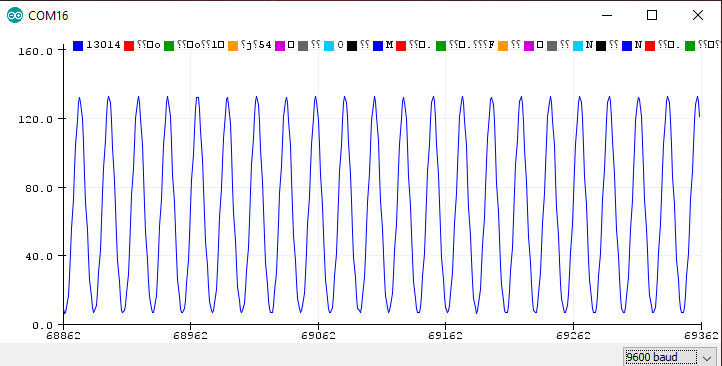A 1 Hz square wave:Finally, a 10 Hz triangular wave:I hope this tutorial has helped you build your own Arduino signal generator. For comments, questions, or suggestions, kindly drop a comment below!

## Watchdog Timer on Arduino Tutorial

In Arduino programming, we learn about functions setup() and loop(). Any code inside setup() executes …

This site uses Akismet to reduce spam. Learn how your comment data is processed.